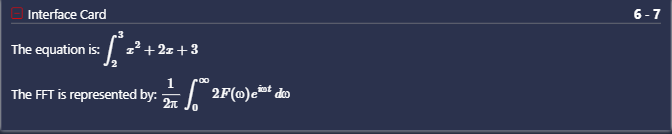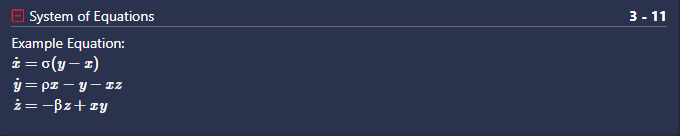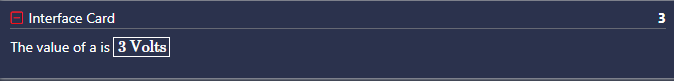# Overview

Artemis also supports the use of LaTeX commands inside documentation blocks. You may use LaTeX in documentation blocks with the following format: $$LATEX_COMMAND$$

# Examples

### Example 1: Using LaTeX with @doc anchor

# @doc The equation is: $$\int_{2}^{3} x^2 + 2x + 3$$
# @doc The FFT is represented by: $$\frac{1}{2\pi} \int_{0}^{\infty} 2F(\omega) e^{i\omega t}\, d\omega$$### Example 2: Using LaTeX with @blockdoc anchor

# @card title="System of Equations"
''' @blockdoc
Example Equation:
\begin{align} \dot{x} & = \sigma(y-x) \\ \dot{y} & = \rho x - y - xz \\ \dot{z} & = -\beta z + xy \end{align}
'''### Example 3 Using LaTeX to box in important pieces of documentation

a = 3
# @doc The value of a is $$\framebox{<<a>> Volts}$$